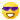Truncated Icosahedron - Soccer Ball

•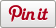• Sharebar
•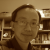Quite a few geometry software can produce complicated geometrical objects. The Platonic solids and Archimedian solids for example, can be produced using dynamic geometry systems (DGS). If so, the process would be brilliant but complicated ways of formal Euclidean  reasoning. In VRMath2, similar reasoning may be used, but because it has a Logo programming or turtle geometry capability, most of these complicated geometrical solids can actually be constructed by moving locations and turning directions.

In order to construct a soccer ball or a truncated icosahedron using Logo or turtle geometry way, it is necessary to know the distance to move and degrees to turn. Upon reading the information on Wikipedia about truncated icosahedron, I found that all edges are the same length, and there are two useful angles for the construction of this truncated icosahedron. These two angles are the two dihedral angles:

• bewteen two hexagonal faces: 138.189685 degrees (6-6)
• between hexagonal and pentagonal faces: 142.62 degrees (6-5)

With the above information, I was able to move and turn the turtle in 3D space to construct a soccer ball as below.

The Logo program is as below, but I believe it can be even simpler.

Please leave a comment below and let me know what you think or your questions.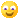In VRMath2 Editor, if "show everything in space"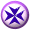icon is clicked, the center of rotation will be calculated automatically.